U.S. Department of Transportation
1200 New Jersey Avenue, SE
Washington, DC 20590
202-366-4000

Federal Highway Administration Research and Technology
Coordinating, Developing, and Delivering Highway Transportation Innovations

 REPORT This report is an archived publication and may contain dated technical, contact, and link information
 Federal Highway Administration > Publications > Research Publications > 14093 > Appendix A. Data Plots
 Publication Number:  FHWA-HRT-14-093    Date:  December 2014
 Publication Number: FHWA-HRT-14-093 Date: December 2014

# Interlaboratory Variability of Slip Coefficient Testing for Bridge Coatings

## APPENDIX A. DATA PLOTS

This appendix contains the load versus slip plots for all specimens tested as part of the interlaboratory variability study. For each coating series, the plots are scaled uniformly for the three or four labs participating to highlight the differences between individual laboratory practice in conducting the RCSC test. Lab 4 only participated in half of the coating series.

For some graphs, the individual data plots within them may be so close to each other that it is difficult to discern one line from the other. To assist readers, a black dashed line has been drawn intersecting all plots at one section. The dashed line is connected to a leader line that connects to the legend. The order of the legend from top to bottom correlates to the order in which the dashed line intersects the individual plots. The intersected plot furthest from the leader is the top entry in the legend, and the intersection closest to the leader is at the bottom of the legend.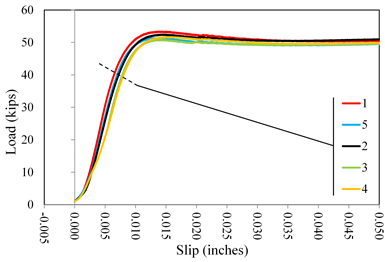Figure 10. Graph. Load versus slip displacement curves for lab 1 coating A1.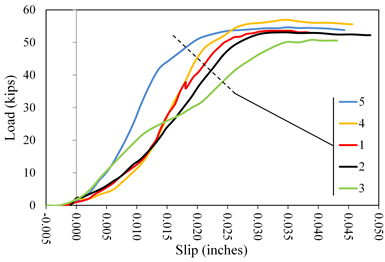Figure 11. Graph. Load versus slip displacement curves for lab 2 coating A1.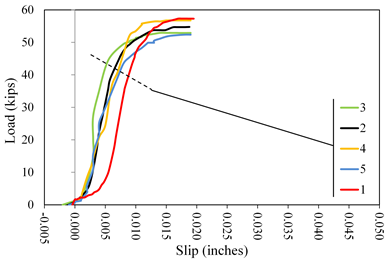Figure 12. Graph. Load versus slip displacement curves for lab 3 coating A1.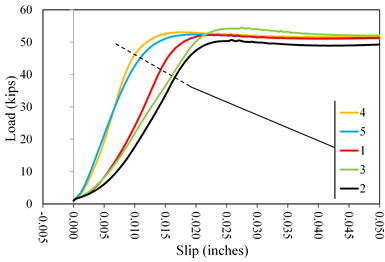Figure 13. Graph. Load versus slip displacement curves for lab 1 coating A2.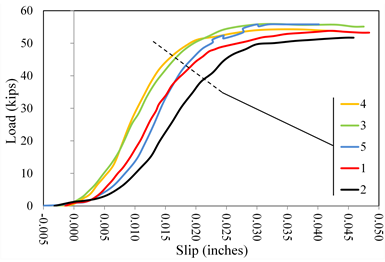Figure 14. Graph. Load versus slip displacement curves for lab 2 coating A2.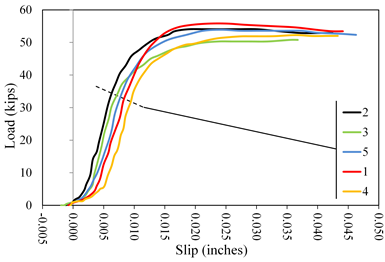Figure 15. Graph. Load versus slip displacement curves for lab 3 coating A2.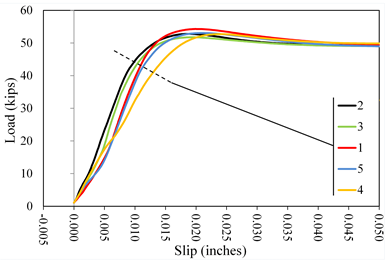Figure 16. Graph. Load versus slip displacement curves for lab 4 coating A2.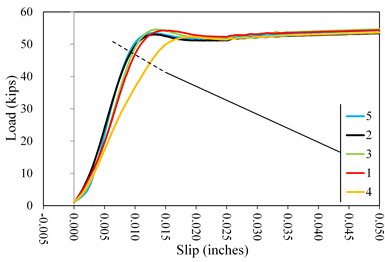Figure 17. Graph. Load versus slip displacement curves for lab 1 coating B1.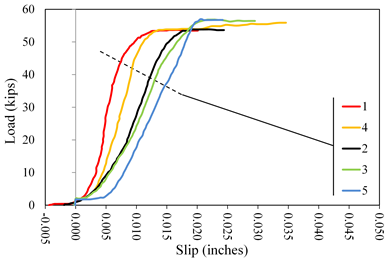Figure 18. Graph. Load versus slip displacement curves for lab 2 coating B1.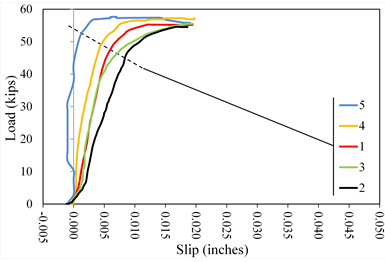Figure 19. Graph. Load versus slip displacement curves for lab 3 coating B1.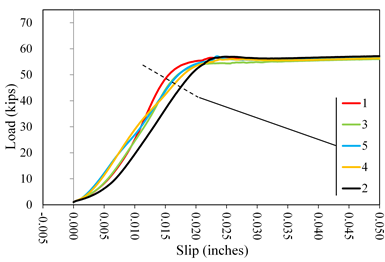Figure 20. Graph. Load versus slip displacement curves for lab 1 coating B2.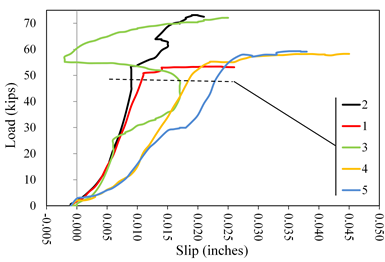Figure 21. Graph. Load versus slip displacement curves for lab 2 coating B2.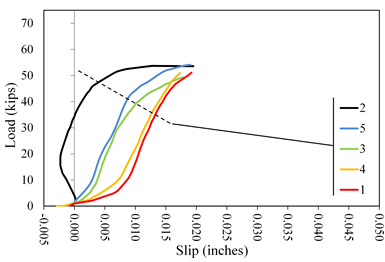Figure 22. Graph. Load versus slip displacement curves for lab 3 coating B2.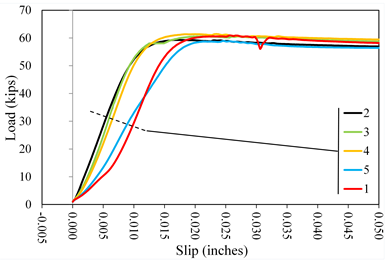Figure 23. Graph. Load versus slip displacement curves for lab 1 coating C1.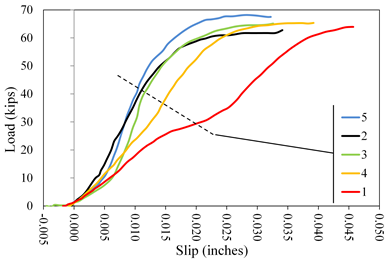Figure 24. Graph. Load versus slip displacement curves for lab 2 coating C1.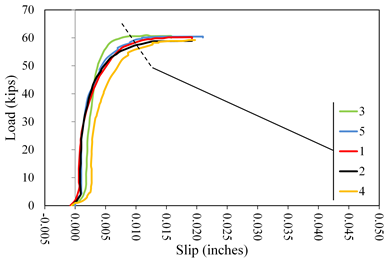Figure 25. Graph. Load versus slip displacement curves for lab 3 coating C1.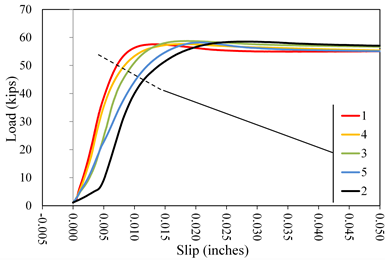Figure 26. Graph. Load versus slip displacement curves for lab 4 coating C1.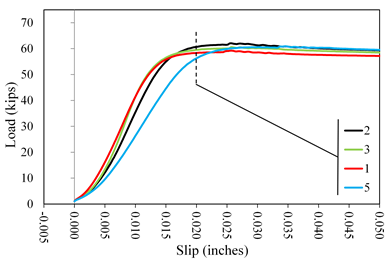Figure 27. Graph. Load versus slip displacement curves for lab 1 coating C2.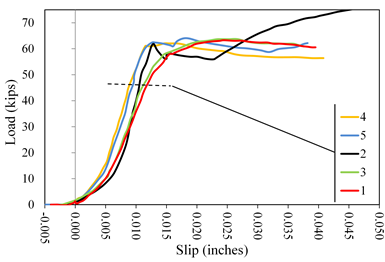Figure 28. Graph. Load versus slip displacement curves for lab 2 coating C2.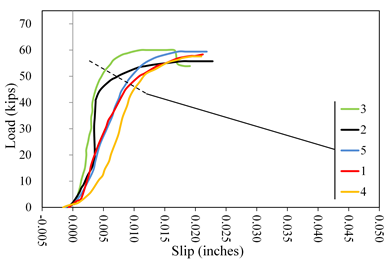Figure 29. Graph. Load versus slip displacement curves for lab 3 coating C2.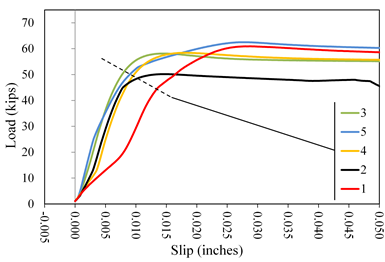Figure 30. Graph. Load versus slip displacement curves for lab 4 coating C2.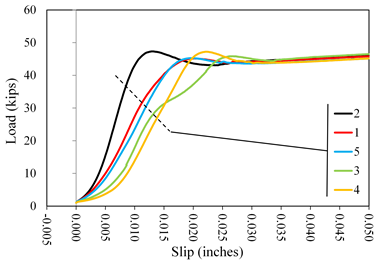Figure 31. Graph. Load versus slip displacement curves for lab 1 coating D1.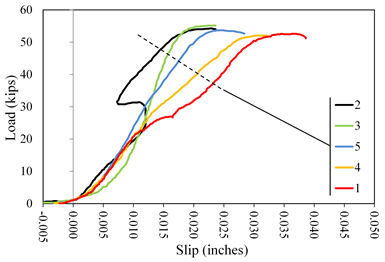Figure 32. Graph. Load versus slip displacement curves for lab 2 coating D1.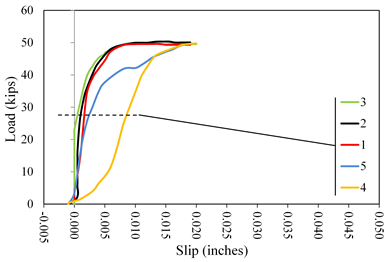Figure 33. Graph. Load versus slip displacement curves for lab 3 coating D1.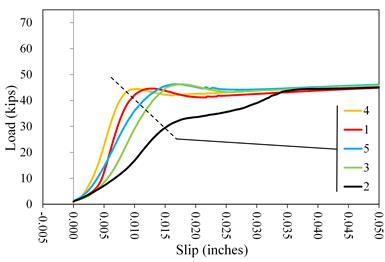Figure 34. Graph. Load versus slip displacement curves for lab 1 coating D2.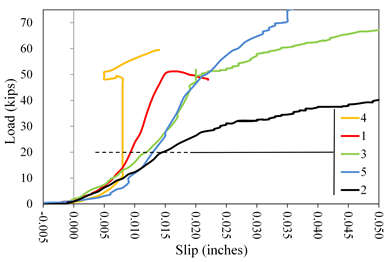Figure 35. Graph. Load versus slip displacement curves for lab 2 coating D2.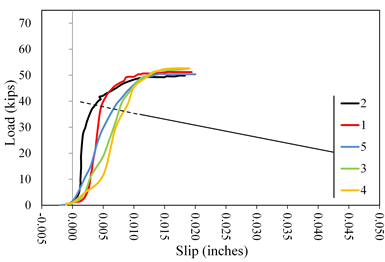Figure 36. Graph. Load versus slip displacement curves for lab 3 coating D2.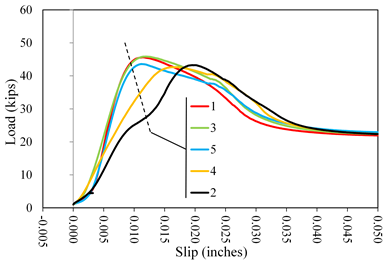Figure 37. Graph. Load versus slip displacement curves for lab 1 coating E1.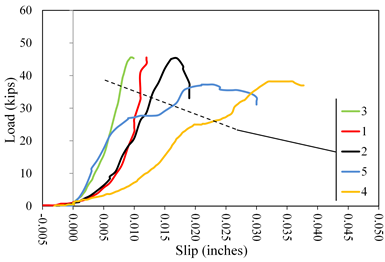Figure 38. Graph. Load versus slip displacement curves for lab 2 coating E1.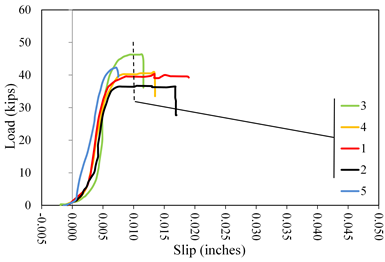Figure 39. Graph. Load versus slip displacement curves for lab 3 coating E1.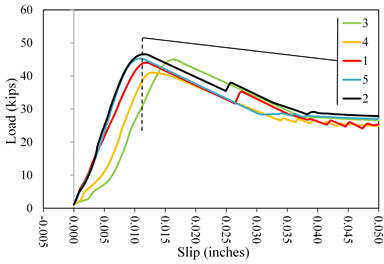Figure 40. Graph. Load versus slip displacement curves for lab 4 coating E1.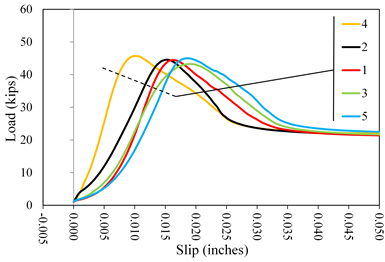Figure 41. Graph. Load versus slip displacement curves for lab 1 coating E2.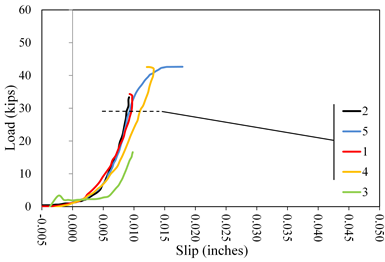Figure 42. Graph. Load versus slip displacement curves for lab 2 coating E2.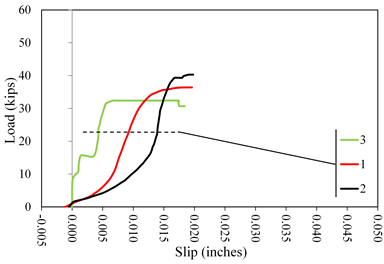Figure 43. Graph. Load versus slip displacement curves for lab 3 coating E2.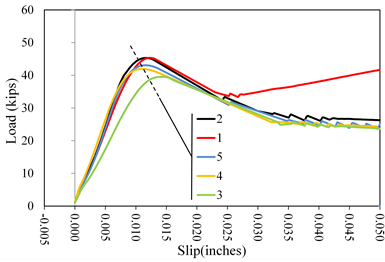Figure 44. Graph. Load versus slip displacement curves for lab 4 coating E2.